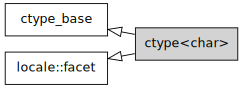/C++

# std::ctype<char>

Defined in header `<locale>`
```template<>
class ctype<char>;```

This specialization of `std::ctype` encapsulates character classification features for type `char`. Unlike general-purpose `std::ctype`, which uses virtual functions, this specialization uses table lookup to classify characters (which is generally faster).

The base class `std::ctype<char>` implements character classification equivalent to the minimal "C" locale. The classification rules can be extended or modified if constructed with a non-default classification table argument, if constructed as `std::ctype_byname<char>` or as a user-defined derived facet. All `std::istream` formatted input functions are required to use `std::ctype<char>` for character classing during input parsing.Inheritance diagram.

### Member types

Member type Definition
`char_type` `char`

### Member functions

 (constructor) constructs a new `std::ctype` facet (public member function) (destructor) destructs a `std::ctype` facet (protected member function) table obtains the character classification table (public member function) classic_table [static] obtains the "C" locale character classification table (public static member function) is classifies a character or a character sequence, using the classification table (public member function) scan_is locates the first character in a sequence that conforms to given classification, using the classification table (public member function) scan_not locates the first character in a sequence that fails given classification, using the classification table (public member function) toupper invokes `do_toupper` (public member function of `std::ctype`) tolower invokes `do_tolower` (public member function of `std::ctype`) widen invokes `do_widen` (public member function of `std::ctype`) narrow invokes `do_narrow` (public member function of `std::ctype`)

### Protected member functions

 do_toupper [virtual] converts a character or characters to uppercase (virtual protected member function of `std::ctype`) do_tolower [virtual] converts a character or characters to lowercase (virtual protected member function of `std::ctype`) do_widen [virtual] converts a character or characters from `char` to `charT` (virtual protected member function of `std::ctype`) do_narrow [virtual] converts a character or characters from `charT` to `char` (virtual protected member function of `std::ctype`)

### Member objects

 static std::locale::id id [static] id of the locale (public static member constant) static const std::size_t table_size [static] size of the classification table, at least 256 (public static member constant)

## Inherited from std::ctype_base

### Member types

Type Definition
`mask` unspecified bitmask type (enumeration, integer type, or bitset)

### Member constants

 space [static] the value of `mask` identifying whitespace character classification (public static member constant) print [static] the value of `mask` identifying printable character classification (public static member constant) cntrl [static] the value of `mask` identifying control character classification (public static member constant) upper [static] the value of `mask` identifying uppercase character classification (public static member constant) lower [static] the value of `mask` identifying lowercase character classification (public static member constant) alpha [static] the value of `mask` identifying alphabetic character classification (public static member constant) digit [static] the value of `mask` identifying digit character classification (public static member constant) punct [static] the value of `mask` identifying punctuation character classification (public static member constant) xdigit [static] the value of `mask` identifying hexadecimal digit character classification (public static member constant) blank [static] (C++11) the value of `mask` identifying blank character classification (public static member constant) alnum [static] `alpha | digit` (public static member constant) graph [static] `alnum | punct` (public static member constant)

### Example

The following example demonstrates modification of ctype<char> to tokenize comma-separated values.

```#include <iostream>
#include <vector>
#include <locale>
#include <sstream>

// This ctype facet classifies commas and endlines as whitespace
struct csv_whitespace : std::ctype<char> {
{
// make a copy of the "C" locale table
static std::vector<mask> v(classic_table(), classic_table() + table_size);
v[','] |=  space;  // comma will be classified as whitespace
v[' '] &= ~space;      // space will not be classified as whitespace
return &v;
}
csv_whitespace(std::size_t refs = 0) : ctype(make_table(), false, refs) {}
};

int main()
{
std::string in = "Column 1,Column 2,Column 3\n123,456,789";
std::string token;

std::cout << "default locale:\n";
std::istringstream s1(in);
while(s1 >> token)
std::cout << "  " << token << '\n';

std::cout << "locale with modified ctype:\n";
std::istringstream s2(in);
s2.imbue(std::locale(s2.getloc(), new csv_whitespace));
while(s2 >> token)
std::cout << "  " << token<< '\n';
}```

Output:

```default locale:
Column
1,Column
2,Column
3
123,456,789
locale with modified ctype:
Column 1
Column 2
Column 3
123
456
789```